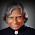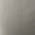Practice is the key to perfection in Quant, so brush up your skills and test yourself with these 15 questions of Quantitative Aptitude for NICL AO Mains 2017.

Directions (1 – 5): Solve the following equations and compare the solutions with the given options:
(a) p≤ q
(b) p≥ q
(c) p< q
(d) p> q
(e) p = q or relationship cannot be established.

Q6. The vehicle of Mr. Ghosh needs 30% more fuel at the speed of 75 kmph than it needs at the speed of 50 kmph. At a speed of 50 kmph, Mr. Ghosh can go to a distance of 195 kms. At the speed of 75 kmph, what distance he will travel?
(a) 125 km
(b) 150 km
(c) 140 km
(d) 175 km

Q7. A shopkeeper sells goods on 20% profit. When he purchases on 10% more and sells it on 25% profit then he gets Rs. 14 more. What will be cost Price?
(a) Rs. 80
(b) Rs. 100
(c) Rs. 125
(d) Rs. 105
(e) None of these

Q8. A man sells two horses for Rs. 1850. Cost price of first horse is equal to selling price of the second horse. If first is sold at 15% loss and second is sold at 25% gain, what is his total loss or gain?
(a) Rs. 100
(b) Rs. 75
(c) Rs. 50
(d) Rs. 80

Q9. If a discount of 10% is given on MP, Mohan gets 20% profit. What will be his profit percent if he offers a discount of 20% on the same article?

Q10.A solid diamond falls and breaks into three pieces whose weights are in 2 : 3 : 4. The value of each piece is directly proportional to the square of their weights. Given that the value of solid diamond is Rs. 24300. What was his loss due to breakage?
(a) Rs. 1,00,00
(b) Rs. 1,56,00
(c) Rs. 1,46,00
(d) Rs. 1,50,00
(e) None of these

Direction (11 – 15): What will come in place of question-mark (?) in the following question?You may also like to Read:

1.please elaborate question no. 6 solution

2.3.This comment has been removed by the author.

4.hello priyanka,
in question no. 6,we have equal patrol for two cases-when you go with-
(1) 50 kmph (2) 75 kmph

they give distance for 50 kmph speed i.e. 195 km
here they give a hint that needs 30% more fuel at the speed of 75 kmph.

case 1. 50 kmph - x patrol for =195
case 2. 75 kmph- x1.3 patrol for =195
now u can simplify value of x which is same for both case

5.Thank you jack